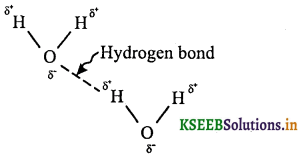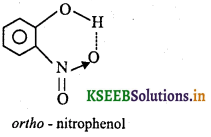# 1st PUC Chemistry Question Bank Chapter 4 Chemical Bonding and Molecular Structure

Students can Download Chemistry Chapter 4 Chemical Bonding and Molecular Structure Questions and Answers, Notes Pdf, 1st PUC Chemistry Question Bank with Answers helps you to revise the complete Karnataka State Board Syllabus and to clear all their doubts, score well in final exams

## Karnataka 1st PUC Chemistry Question Bank Chapter 4 Chemical Bonding and Molecular Structure

Question 1.
What is chemical bond?
The attractive force which holds various constituents (ions, atoms, molecules, etc) together in different chemical species is called a chemical bond.

Kossel – Lewis Approach to Chemical Bonding

Question 1.
What are valence electrons?
The electrons that participate in the formation of chemical bonds are called valence electrons.

Question 2.
What is Lewis symbol?
Lewis symbol is the symbol of an element surrounded by ‘dots’ to represent the number of valence electrons.Question 3.
Write the Lewis symbols for second period elements (Li, Be, B, C, N, O, F and Ne).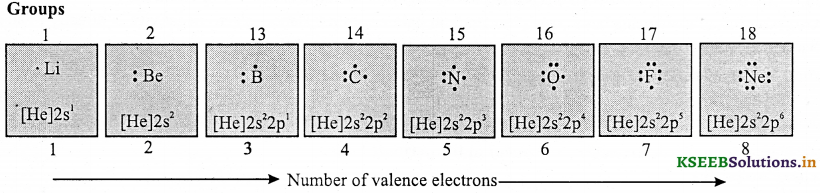Question 4.
State the postulate of Lewis with respect to chemical bond and stability of an atom.
Lewis postulated that “atoms achieve the stable octet when they are linked by chemical bonds”.

Question 5.
State octet rule.
The tendency of atoms in a molecule to have eight electrons in their valence shell (ns2np6) is known as octet rule.

Question 6.
Give the significance of Lewis symbols.

• The numbers of dots around the symbol represents the number of valence electrons.
• The number of valence electrons helps to calculate the group valency of the element.

Question 7.
How do you calculate the group valence of the elements by using symbols?
The group valence of the element is equal to number of dots in Lewis symbols or 8 minus the number of dots or valence electrons.

Question 8.
What is covalent bond? Explain the formation of covalent bond by taking one example.
A covalent bond is formed when the atoms of same or different elements combine by mutual sharing of electrons.
Example
1. Formation of single covalent bond in chlorine: Single covalent bond is formed by sharing of one pair of electrons between two atoms.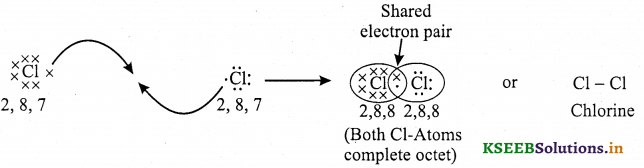2. Formation of double covalent bond in carbon dioxide: Double bond is formed by the sharing of two pairs of electrons between two atoms.3. Formation of triple covalent bond in acetylene: Triple bond is formed by the sharing of three pairs of electrons between two atoms.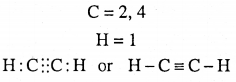Question 9.
Write the Lewis symbols of H2, O2, O3, NF3, CO2-3 , HNO3.Question 10.
Write the Lewis symbols of CO, NO2, CCl4 and H2O.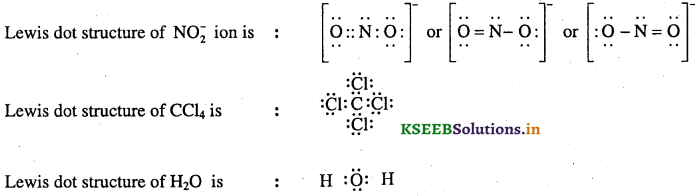Question 11.
Define formal charge. Give the equation to calculate the formal charge.
Formal charge is defined as “the difference between the valence electrons in the neutral atom and the number of électrons assigned to that atom in a Lewis structure.”
Formal Charge equation:Question 12.
Give the importance of formal charge.
Formal charge helps in the selection of the lowest energy structure from a number of possible Lewis structures for a given species. Generally the lowest energy structure is the one with the smallest formal charge on the atoms. The formal charge is a factor based on a pure covalent view of bonding in which electron pairs are shared equally by neighbouring atoms.

Question 13.
Calculate the formal charge on each oxygen atom in ozone.
The Lewis structure of O3 (structure I) may be drawn as:
Formal charge of O marked 1 = 6 – $$\frac { 1 }{ 2 }$$ × (6) – 2 = + 1
Formal charge of O marked2 = 6 – $$\frac { 1 }{ 2 }$$ × (4) -4 = 0
Formal charge of O marked 3 = 6 – $$\frac { 1 }{ 2 }$$ × (2) – 6 = -l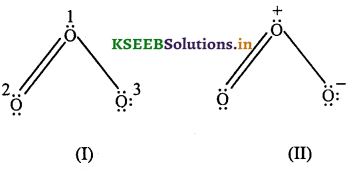Hence, we represent O3 along with the formal charges as (structure II)Question 14.
Give the limitations of the octet rule.
(a) It is based upon the inert nature of noble gases. However, some noble gases like xenon and krypton form compounds such as XeF2, KrF2, etc.
(b) The octet rule cannot be applied to the elements in and beyond the third period of the periodic table. The elements present in these periods have more than eight valence electrons around the central atom. For example: PF5, SF6, etc.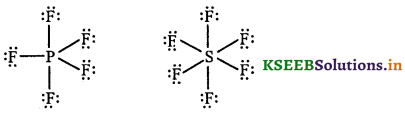(c) The octet rule is not satisfied for all atoms in a molecule having an odd number of electrons. For example, NO and NO2 do not satisfy the octet rule.
(d) This rule cannot be applied to those compounds in which the number of electrons surrounding the central atom is less than eight. For example, LiCl, BeH2, AlCl3 etc. do not obey the octet rule.
(e) The rule failed to predict the shape and relative stability of molecules.
(f) This theory does not account for the shape of molecules.

Question 15.
Give one example each for a molecule with
(i) Odd number of electrons on the central atom and
(ii) Expanded octet.
(i) NO or NO2
(ii) PF5 and SF6

Ionic Bond or Electrovalent Bond

Question 1.
What is ionic bond? Explain the formation of ionic bond with an example.
The bond formed by the electrostatic force of attraction between positive and negative ions is called ionic bond.
Ionic bond is formed by the transfer of one or more electrons from one atom to another atom.
Example 1: Formation of NaCl from sodium and chlorine as follows.Question 2.
What are the conditions to form ionic bond?
Ionic bond is formed between the atoms having
(a) low ionisation enthalpies (Alkali metals)
(b) high negative value of electron gain enthalpy (Halogens).
(c) High lattice enthalpy. (Lattice energy)

What type of bond is present in CaF2?
Ionic bondQuestion 3.
What is lattice enthalpy of ionic crystal? Give the relation between lattice enthalpy and stability of ionic crystal.
The lattice enthalpy of ionic crystal is defined as the energy required to completely separate one mole of a solid ionic compound into gaseous constituent ions.
Higher the lattice energy, higher the stability of ionic compound.

Bond Parameters

Question 1.
Define bond length.
It is defined as the equilibrium distance between the nuclei of two bonded atoms in a molecule.

Question 2.
It is defined as half of the distance between two similar atoms linked by a covalent bond in the same molecule.

Quetion 3.
It is defined as half of the distance between two similar atoms in separate neighbouring molecules in a solid.Question 4.
What does vander Wall’s radius represents?
It represents the overrall size of an atom.

Question 5.
Define bond angle. What is the bond angle in water molecule?
The angle between the two orbitais containing bonding electron pairs around the central atom in a molecule is called bond angle.
The bond angle in water molecule is 104.5° or 104°31′.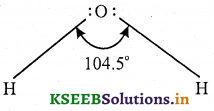Question 6.
Define bond enthalpy. How do you obtain bond enthalpy in poiy atomic molecules?
Bond enthalpy is defined as the amount of energy required to break one mole of bonds of a particular type
between two atoms in a gaseous state.The bond enthalpy of hydrogen molecule is 435.8 kJ.
In polyatomic molecules, bond enthalpy is calculated interms of mean or average bond enthalpy. It is obtained by dividing total bond dissociation enthalpy by the number of bonds broken. This is because bond enthalpy of
different types of bonds in polyatomic molecule is not same.
For example, average bond enthalpy in water molecule is
H2O(g) → H(g) + OH(g) Δa H1 = 502 KJ mol-1
OH(g) → O(g) + H(g) Δa H2 = 427 KJ mol-1
Average bond enthalpy of water molecule = $$\frac{502+427}{2}$$ = 464.5 KJ mol-1.Question 7.
What is bond order?
Bond order is the number of covalent bonds between the two atoms in a molecule
Example:
H2 : H – H (single bond) ∴ bond order = 1
O2: O = O (double bond) ∴ bond order 2
N2 : N ≡ N (triple b ond) ∴ bond order = 3
Note:

• Isoelectronic molecules and ions have identical bond orders. Example: F2 and O2-2 have bond order 1.
• N2, CO and NO+ have bond order 3
• Higher the bond order, higher the bond enthalpy, lower the bond length and higher the stability.

Question 8.
How do you explain the structure of molecules by resonance?
According to the concept of resonance, whenever a single Lewis structure cannot describe a molecule accurately, a number of structures with similar energy, position of nuclei, bonding and non bonding pairs of electrons are taken as the canonical structures of the hybrid which describes the molecule accurately.

Question 9.
What is resonance hybrid?
The weighted average contributing canonical structures is called resonance hybrid. Resonance is represented by double headed arrow (↔).

Question 10.
Write the resonance structures and resonance hybrid of ozone molecule.
Structures I and II are resonance Structures of O3. structure III is resonance hybrid of ozone.Question 11.
Write the resonance structures of CO32- ion.
The resonance structures of CO32- ion can be represented as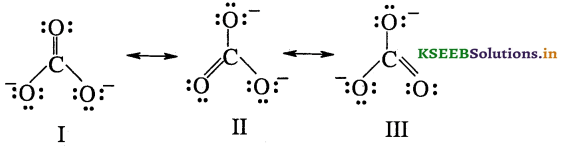Each structure has two C – O single bonds and one C = O double bond. It represents unequal number of bonds between C and O atoms. But experiment has shown that all the three C – O bonds are of equal length. Hence carbonate ion is best described by resonance hybrid of canonical forms I, II and III.Question 12.
Explain the resonance structure of CO2 molecule.
The resonance structures of CO2 molecule can be represented asThe carbon – oxygen bond lengths in CO2 (115 pm) lie between the values for C = O and C ≡ O .Hence the structure of CO2 is best described as a hybrid of the canonical forms I, II and III.

Question 13.
What is non polar covalent bond?
The covalent bond in which the atoms having same electronegativity are equally attracted with respect to each other is called non polar covalent bond.
Examples. H2, O2, Cl2, N2 and F2.

Question 14.
What is polar covalent bond?
The covalent bond in which the shared electron pair between the two different atoms gets displaced more towards high electronegative atom induces the polarity. This bond is called polar covalent bond.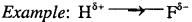Question 15.
Define dipole moment (µ). What is its unit?
It is defined as “the product of the magnitude of the charge and the distance between the centres of positive and negative charge.”
Dipole moment (µ) = Charge (Q) × Distance of separation (γ)
The unit of dipole moment is Debye. (D)

Question 16.
What is the value of 1 Debye in coloumb metre?
ID = 3.33564 × 10-30Cm ;

Question 17.
Is dipole moment is vector or scalar quantity?
Vector quantity.

Question 18.
On what factors does the dipole moment of polyatomic molecules depend?

• Bond dipoles
• Spatial arrangement of various bonds in the molecule.

Question 19.
What is the net dipole moment of water and show bond dipoles in its structure?
Dipole moment of water, µ = 1.850D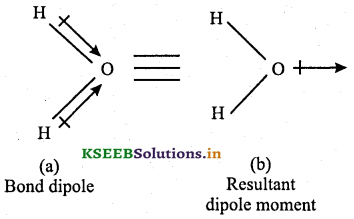Question 20.
What is the resultant dipole moment of water in coulomb meter?
µ = 1.85 × 3.33564 × 10-30 = 6.17 × 10-30 Cm.

Question 21.
What is the dipole moment in BeF2? Show bond dipoles in its molecule.
Dipole moment of BeF2 is zero, since it is linear molecule.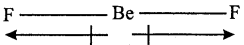Question 22.
Dipole moment in BF3 molecule is Zero. Explain.
The bond dipoles in BF3 molecule can be written as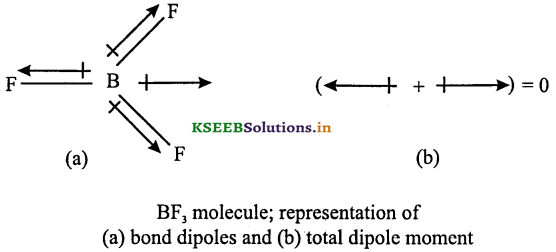Although the B – F bonds are oriented at angle of 120° to one another, the three bond moments give a net sum of zero because the resultant of any two is equal and opposite to the third.

Question 23.
The resultant dipole moment of NH3 is greater than NF3. Give reasons.
The net dipole moment of NH3 is greater than NF3 because in NH3, the orbital dipole due to lone pair is in the same direction as the resultant dipole moment of the N – H bonds.

But in NF3, the orbital dipole is in the direction opposite to the resultant dipole moment of the three N – F bonds. The orbital dipole because of the lone pair decreases the effect of the resultant N – F bond moments, which results in the low dipole moment of NF3 as represented as: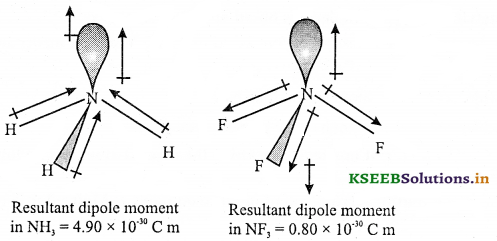Question 24.
Explain Fajan’s rules.
The partial covalent character of ionic bonds was discussed by Fajan according to the following rules:

• Smaller the size of the cation and larger the size of the anion, greater the covalent character of an ionic bond.
• The greater the charge on the cation, greater the covalent character of the ionic bond.
• For cations of the same size and charge, the one with electronic configuration (n – l)dn ns° (typical of transition metals) is more polarising than the one with a noble gas configuration, ns2np6 (typical alkali and alkaline earth metal cations).

The cation polarises the anion, pulling the electronic charge toward itself and thereby increasing the electronic charge between the two. The polarising power of the cation, the polarisability of the anion and the extent of distortion (polarisation) of anion are the factors, which determine the percentage of covalent character of the ionic bond.

Question 25.
What are the factors which determine the percent covalent character of the ionic bond?
Ans

• Size of cation and anion
• Charge on the cation

Question 26.
Arrange the following basic compounds in the increasing order of covalent character: NaCl, KCl, LiCl.
KCl < NaCl < LiCl

Valence Shell Electron Pair Repulsion Theory

Question 1.
Give the main postulates of VSEPR theory.
Postulates
1. The geometry of a molecule depends upon the total number of valence shell electron pairs (bonded or not bonded) present around the central atom and their repulsion due to relative sizes and shapes.

2. Pairs of electrons in the valence shell repel one another since their electron clouds are negatively charged.

3. These pairs of electrons tend to occupy the positions in space that minimise repulsion and thus maximise distance between them.

4. If the central atom is surrounded by lone pairs (lp) as well as bond pairs (bp), then the molecule will have a distorted geometry.

5. The relative order of repulsion between electron pairs is as follows: lp – lp > lp – bp > bp – bp.

6. A lone pair is concentrated around the central atom while a bond pair is pulled out between two bonded atoms. As a result repulsion becomes greater when a lone pair is involved.Question 2.
Give the geometrical shapes of molecules based on VESPR theory.
Geometrical shapes of molecules can be predicted by using VSEPER theory by dividing the molecules into two categories as

• Molecules in which the central atom has no lone pair
• Molecules in which the central atom has one or more lone pairs.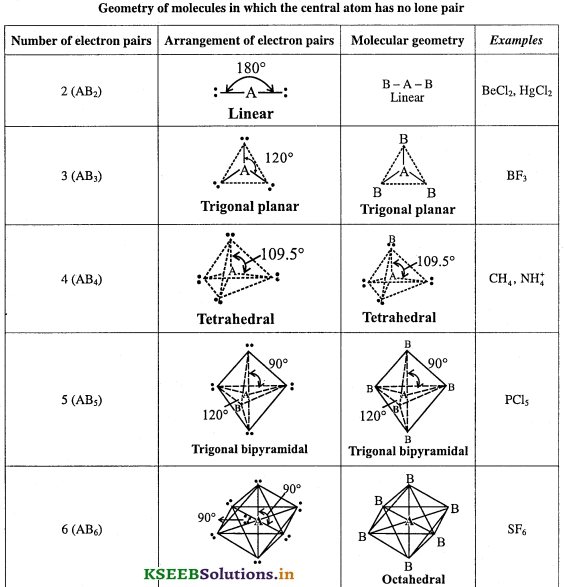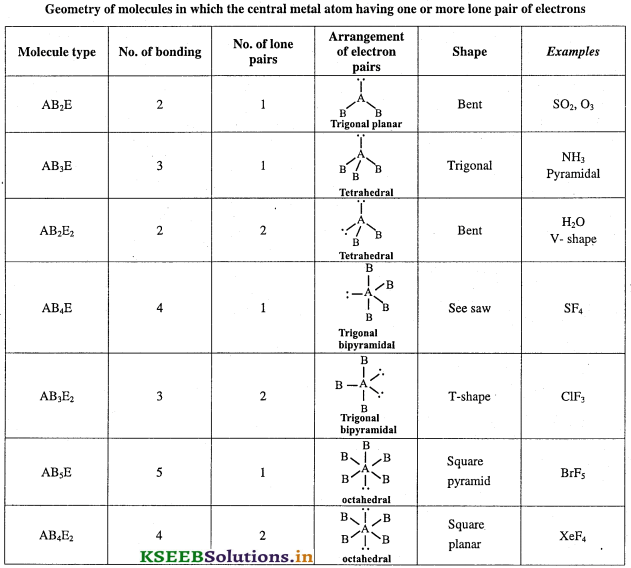Geometry of molecules in which the central atom has no lone pairQuestion 3.
Predict the shapes of BeCl2, BF3, CH4, NH3 and H2O molecules on the basis of VSEPR theory.
Beryllium chloride (BeCl2) : The BeCl2 molecule has a Lewis dot structure as shown. The central Be atom has two bond pairs in its outer shell. Repulsion between these two pairs causes the molecule to be linear. This results in the molecule having a linear geometry.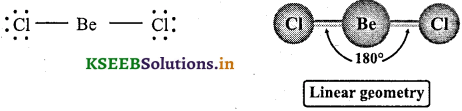Boron triflouride (BF3): In BF3 molecule, the Boron atom has atomic number 5 and has electronic configuration of 1s22s22p1 Thus, Boron has 3 valence electrons. These electrons are shared mutually with three fluorine atoms to form three B – F bonds. So, in BF3, Boron has no lone pair. Hence BF3 has trigonal planar geometry. .
Shape: Trigonal planar
Number of bond pairs = 3
Number of lone pairs = 0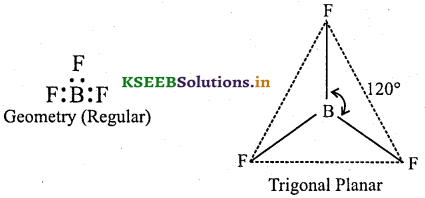Total number of electron pairs = bp + lp = 3 + 0 = 3

Methane (CH4): In CH4 molecule, the Carbon atom has atomic number 6 and has valence shell electronic configuration of 2s2 2p2. Thus, Carbon has 4 valence electrons. These electrons are shared mutually with four hydrogen atoms to form four C – H bonds. So, in CH4, carbon has no lone pair. Hence CH4 has regular tetrahedral geometry.
Shape: Tetrahedral
Number of bond pairs = 4
Number of lone pairs = 0Total number of electron pairs = bp + Ip = 4 + 0 = 4 Regular tetrahedral

Ammonia (NH3): In NH3 molecule, the nitrogen atom has atomic number 7 and has electronic configuration of 1s2 2s2 2p3. Thus, Nitrogen has 5 valence electrons. Out of these, 3 electrons are shared mutually with three hydrogen atoms to form three N – H bonds and the remaining 2 electrons exist as a lone pair. So, in NH3, Nitrogen has one lone pair of electrons.
Shape: Pyramidal
Number of bond pairs = 3
Number of lone pairs = 1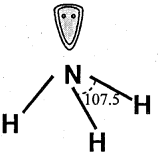Total number of electron pairs = bp+ lp = 3 + 1= 4.
According to VSEPR theory, NH3 should have tetrahedral shape due to presence of 4 electron pairs. The presence one lone pair on nitrogen causes greater repulsion to the bond pairs. As a result of which the three N – H bonds move slightly closer. So it decreases the normal tetrahedral angle of 109.5° to 107°. Therefore, ammonia has irregular geometry. Since one of the tetrahedral positions is occupied by lone pair, the shape of ammonia molecule is pyramidal.

Water (H2O): In H2O molecule, the Oxygen atom has atomic number 8 and has electronic configuration of 1s2 2s2 2p4. Thus, Oxygen has 6 valence electrons. Out of these, 2 electrons are shared mutually with two hydrogen atoms to form two O – H bonds and the remaining 4 electrons exist as two lone pairs. So, in H2O, Oxygen has two lone pairs of electrons.
Number of bond pairs = 2
Number of lone pairs = 2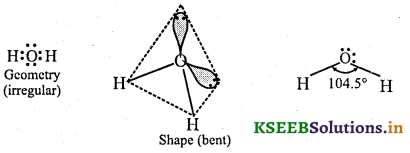Total number of electron pairs = bp+ lp = 2 + 2 = 4
According to VSEPR theory, H2O should have tetrahedral shape due to presence of 4 electron pairs. The presence of two lone pairs of electrons on oxygen causes greater repulsion to the bond pairs. As a result the two O – H bonds move closer. So the normal tetrahedral angle of 109.5° reduced to 104.5°. So, water molecule has irregular geometry. Since two of the tetrahedral positions are occupied by lone pairs, the shape of water molecule is Bent or V-shape.Valence Bond Theory

Question 1.
Give the main postulates of valence bond theory (VBT)

• A covalent bond is formed by the overlapping of two half-filled valence atomic orbitals of two same or different atoms.
• The electrons in the overlapping orbitals get paired and confined between the nuclei of two atoms.
• The electron density between two bonded atoms increases due to overlapping. This confirm stability to the molecule.
• Attraction increases as the distance between the atoms gets closer but nuclear-nuclear repulsion becomes important if the atoms approach too close.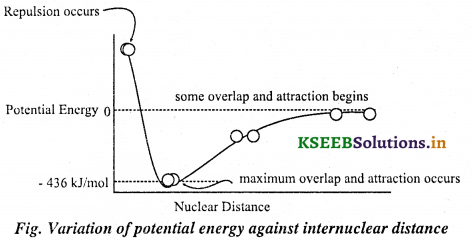• Greater the extent of overlapping, stronger is the bond formed.
• The direction of the covalent bond is along the region of overlapping of the atomic orbitals, i.e., covalent bond is directional.

Question 2.
Give the importance of VBT.
VBT explains the shape, the formation and directional properties of bonds in poly atomic molecules in terms of overlapping and hybridization of atomic orbitals.

Question 3.
Explain overlapping of atomic orbitals.
When orbitals of two atoms come close to form bond, their overlapping may be positive, negative or zero depending upon the sign (phase) and direction of orientation of amplitude of orbital wave function in space.
(a) Positive overlap: Orbitals forming bond should have same sign (phase) and orientation in space. This is called positive overlap.
Examples:(b) Negative overlap: Orbitals forming bond should have opposite sign (out of phase) and orientation in space. This is called negative overlap. Examples: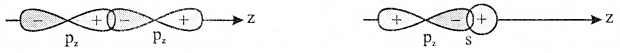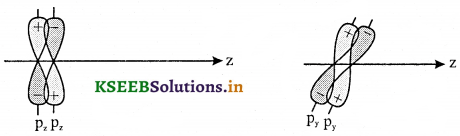(c) Zero over lap: This arises out of phase due to direction of approach.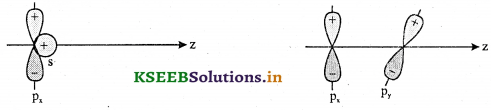Question 4.
Explain types of overlapping and nature of covalent bonds.
Types of bonds
σ – bond: A covalent bond formed by the overlapping of atomic orbitals along the inter nuclear axis is called
σ – bond”. It is a stronger bond and cylindrically symmetrical. Depending on the types of orbitals that overlap, the σ – bond is divided into following types:
σs-s bond: s-s sigma bond is formed by the overlapping of two half filled s orbitals.Example, Formation of H2 molecule

• The electronic configuration of hydrogen atom in the ground state is 1s1
• In the formation of hydrogen molecule, two half-filled 1 s orbitals of hydrogen atoms overlap along the inter- nuclear axis and form σs-s bond.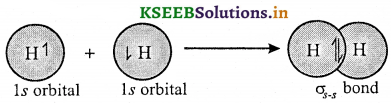σp-p bond: p-p sigma bond is formed by overlapping of two half filled p orbitals.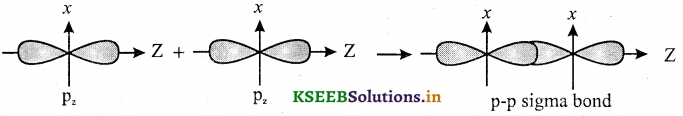Example, Formation of Cl2 molecule

• The electronic configuration of Cl atom in the ground state is [Ne] 3s2 3px2 3py2 3pz1.
• The two half-filled 3pz atomic orbitals of two chlorine atoms overlap along the inter-nuclear axis and form a σp-p bond.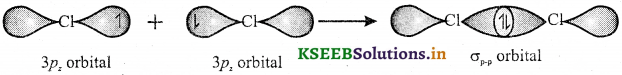3pz orbital 3pz orbital orbital
σs-p bond: s-p sigma bond is formed by overlapping of one half filled s orbital and one half filled p orbitals along the internuclear axis.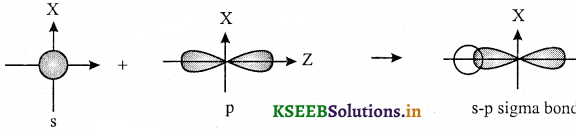Example, formation of HCl molecule

• In the ground state, the electronic configuration of hydrogen atom is lx1.
• And the ground state electronic configuration of Cl atom is [Ne]3x2 3px2 3py2 3pz1.
• The half-filled hv orbital of hydrogen atom overlap with the half-filled 3pz atomic orbital of chlorine atom along the inter-nuclear axis to form a σs-p bond.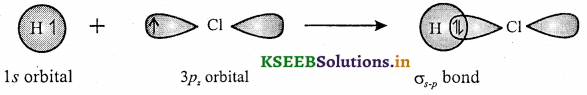π-bond: A covalent bond formed by the sideways overlapping of atomic orbitals is called π-bond. In this bond, the electron density is present above and below the inter nuclear axis. It is relatively a weaker bond since the electrons are not strongly attracted by the nuclei of bonding atoms (extent of overlapping is minimum).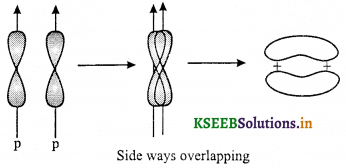Note: The ‘s’ orbitals can only form σ – bonds, whereas the p, d and f – orbitals can form both σ – and π – bonds.Question 5.
Give differences between a and n bonds.

 Sigma (σ) bond Pi (π) bond 1. It is formed by the axial overlapping of the atomic orbitals. 1. It is formed by the sideways overlapping of the atomic orbitals. 2. The overlap is quite effective and the bond is quite strong 2. The overlap is less and the bond is comparatively weak. 3. x-orbitals can take part in the sigma bond formation 3. s-orbitals don’t take part in the π-bond formation. 4. Only one lobe of the p-orbital is involved in the overlap. 4. Both lobes of the p-orbitals are involved in the overlap 5. The electron cloud of the molecular orbital is symmetrical around the internuclear axis. 5. The electron cloud is unsymmetrical. 6. The σ bond can be formed alone 7. The π bond can be formed along with σ bond. 7. Free rotation of the atoms around σ -bond is possible. 8. Free rotation of the atoms around π-bond is not possible

Hybridisation

Question 1.
What is hybridization?
Hybridisation is defined as intermixing of pure atomic orbitals of slightly different energies and different shapes to give equal number of hybrid orbitals of same energies and identical shape. The new orbitals formed are known as hybrid orbitals.

Question 2.
Give salient features of hybridization.

• The number of hybrid orbitals produced is equal to the number of orbitals undergoing hybridization.
• The hybridised orbitals are always equivalent in energy and shape.
• The hybrid orbitals can form stronger bonds as compared to atomic orbitals because they are more directional and hence can overlap to a greater extent.
• The hybrid orbitals are directed in space in some preferred direction to have minimum repulsion between electron pairs and thus a stable arrangement. Therefore, based on the type of hybridization, the geometry and bond angles of a molecule can be predicted.

Question 3.
Give important conditions for hybridization.

• The orbitals present in the valence shell of the atom are hybridised.
• The orbitals undergoing hybridization should have almost equal energy.
• Promotion of electron is not an essential condition to undergo hybridisation.
• It is not necessary that only half filled orbitals participate in hybridisation. The completely filled or even empty orbitals can undergo hybridization provided they have almost equal energy.

Question 4.
Explain sp hybridization by taking BeCl2 as an example.
Intermixing of one ‘s’ and one ‘p’ orbitals of almost equal energy to give two identical and degenerate hybrid orbitals is called ‘sp’ hybridization.

• These sp-hybrid orbitals are arranged linearly and hence bond angle is 180°.
• They possess 50% ‘s’ and 50% ‘p’ character.
• Remaining py and pz unhybrid orbitals lie perpendicular to the plane of sp-orbital.

Example: Formation of BeCl2 molecule
BeCl2 molecule has linear shape.Question 5.
Explain sp hybridization in acetylene (C2H2) molecule.
Molecular formula of ethyne is C2H2. In ethyne, each carbon atom is sp-hybridized. In this way, four sp-orbitals are generated. One sp-orbital of each carbon atom overlap and form a sigma bond between carbon atoms. Remaining one sp-orbital of each carbon atom overlaps with 1s-orbital of hydrogen atom to produce two sigma bonds, py and pz orbital of each carbon overlap sideways and form two pi-bonds between two carbon atoms. Geometry of ethyne molecule is linear in which bond angles are 180°.Question 6.
Explain sp2 hybridisation by taking BCl3 as example.
sp2-hybridization

• Intermixing of one ‘s’ and two ‘p’ orbitals of almost equal energy to give three identical and degenerate hybrid orbitals is known as sp2 hybridization.
• The three sp2 hybrid orbitals are oriented in trigonal planar symmetry at angles of 120° to each other.
• The sp2 hybrid orbitals have 33.3% ‘s’ character and 66.6% ‘p’ character.
• The fourth unhybridised px – orbital lies at right angle to the plane of sp2 – orbitals.Boron trichloride (BCl3)
The electronic configuration of ‘B’ in ground state is 1s2 2s2 2p1 with only one unpaired electron. Since the formation of three bonds with chlorine atoms require three unpaired electrons, there is promotion of one of 2s electrons into the 2p sublevel by absorbing energy. Thus Boron atom gets electronic configuration: 1s2 2s1 2p1x 2p1y in the excited state.

In the excited state, Boron undergoes sp2 hybridization by using a 2s and two 2p orbitals to give three.half- filled sp2 hybrid orbitals which are oriented in trigonal planar symmetry.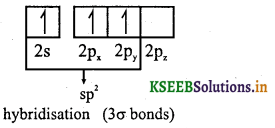Boron forms three σsp-p bonds with three chlorine atoms by using its half-filled sp2 hybrid orbitals. Each chlorine atom uses its half-filled p-orbital for the σ-bond formation.

Thus the shape of BCl3 is trigonal planar with bond angle equal to 120°.Question 7.
Explain sp2 hybridisation in ethylene (C2H4) molecule.
Molecular formula of ethene (ethylene) is C2H4. In ethene, each carbon atom is sp2-hybridized. In this way six sp2-orbitals are generated (three for each carbon atom). One sp2-orbital of each carbon atom by overlapping forms a sigma bond between carbon atoms. Remaining two sp2-orbital of each atom overlap with 1s-orbital of hydrogen atom to produce four sigma bonds. pz unhybrid orbital of each carbon atom by sideways overlapping form a Pi – bond between two carbon atoms. Ethene is a Planar molecule.Question 8.
Explain sp3 hybridisation by taking CH4 as an example.

• In sp3 hybridisation, one ‘s’ and three ‘p’ orbitals of almost equal energy intermix to give four identical and degenerate hybrid orbitals.
• These four sp3 hybrid orbitals are oriented in tetrahedral symmetry with 109°28′ angle or 109.5° as bond.
• The sp3 hybrid orbitals have 25% ‘s’ character and 75% ‘p’ character.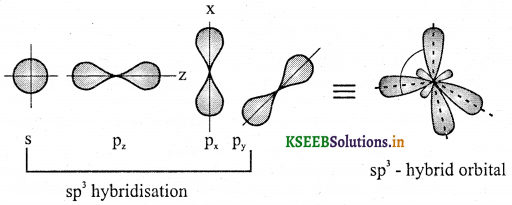Formation of CH4 molecule:
In methane, the central atom is carbon (Z = 6).
Electronic configuration 1s2, 2s2 2px1 2py1.
Electronic configuration in excited state 1s2, 2s1 2px1 2py1 2pz1

Carbon atom in the ground state has only two unpaired electrons but in the excited state one 2s electron is promoted to vacant 2pz orbital. Thus there are four unpaired electrons, one each in 2s, 2px, 2py and 2pz orbitals. These four orbitals hybridize and form four equivalent sp3 hybrid orbitals directed towards the four comers of a regular tetrahedron with bond angle 109°.28′. Each sp3 hybrid orbital, overlaps with 1s orbital of hydrogen atom and forms four C – H bonds in CH4 molecule. Thus each C – H bond involves sp3 – s overlap and methane molecule has tetrahedral structure with H – C – H bond angle 109°.28′.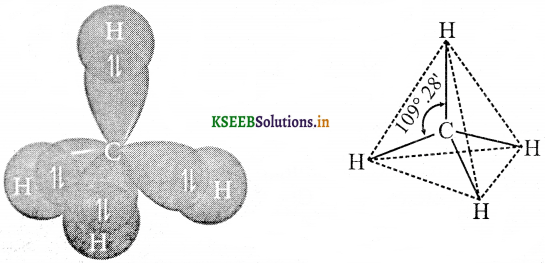Question 9.
Explain sp3 hybridisation in the ethane molecule.
Just like in methane molecule, each carbon atom undergoes sp3 hybridization in the excited state to give four sp3 hybrid orbitals in tetrahedral geometry.
♦ The two carbon atoms form a σsp3– sp3 bond with each other due to overlapping of sp3 hybrid orbitals along the inter-nuclear axis.
♦ Each carbon atom also forms three σsp3 – s bonds with hydrogen atoms.
♦ Thus there is tetrahedral geometry around each carbon with bond Angles equal to 109°.28′.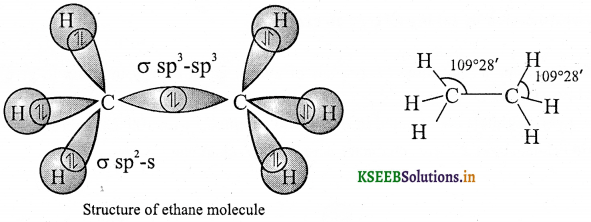Question 10.
Explain sp3d hybridisation in the formation of PCl5.

• The ground state electronic configuration of phosphorus atom is: 1s2 2s2 2p6 3s2 3px1 3py1 3pz13d1
• The formation of PCl5 molecule requires 5 unpaired electrons. Hence the phosphorus atom undergoes excitation to promote one electron from 3s orbital to one of empty 3d orbital.
• Thus the electronic configuiation of Phosphorus in the excited state is 1s2 2s2 2p6 3s1 3px1 3py1 3pz13d1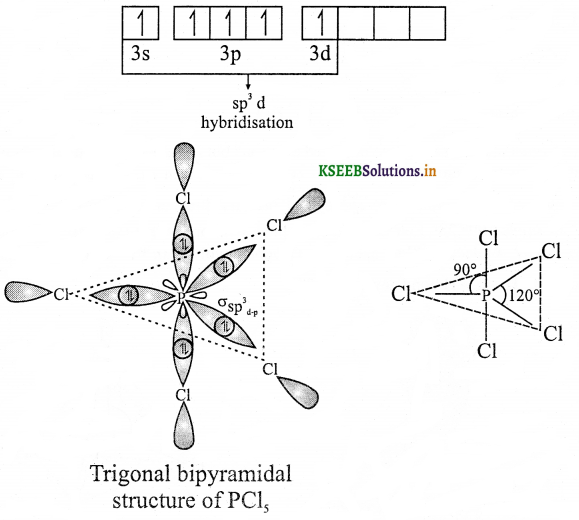• In the excited state, intermixing of one 3s, three 3p and one 3d orbitals to give five half- filled sp3d hybrid orbitals, which are arranged in trigonal bipyramidal geometry i.e., three orbitals are arranged in trigonal planar symmetry, whereas the remaining two are arranged perpendicularly above and below this plane.
• By using these half-filled sp3d orbitals, phosphorus forms five σsp3d bonds with chlorine atoms. Each chlorine atom makes use of half-filled 3Pz orbital for the bond formation.
• The shape of PCl5 molecule is trigonal bipyramidal with 120° and 90° of Cl – P – Cl bond angles.Question 11.
Explain sp3d2 hybridisation in the formation of SF6.

• The electronic configuration of Sulphur in ground state is 1s2 2s2 2p6 3s2 3px1 3py1 3pz1
• In SF6 molecule, there are six bonds formed by sulphur atom. Hence there must be 6 unpaired electrons.
However, there are only 2 unpaired electrons in the ground state of sulphur. Hence it promotes two electrons into two of the 3d orbitals (one from 3s and one from 3px).
• Thus the electronic configuration of sulphur in its excited state is 1s2 2s2 2p6 3s1 3px1 3py1 3pz13d2.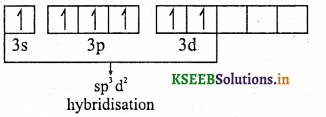• In the excited state, sulphur under goes sp3d2 hybridization by mixing a 3s, three 3p and two 3d orbitals. Thus formed six half-filled sp3d2 hybrid orbitals are arranged in octahedral geometry.
• Sulphur atom forms six σsp3d2-p bonds with 6 fluorine atoms by using these sp3d2 orbitals. Each fluorine atom uses is half-filled 2pz orbitals for the bond formation. SF6 is octahedral in shape with bond angles equal to 90°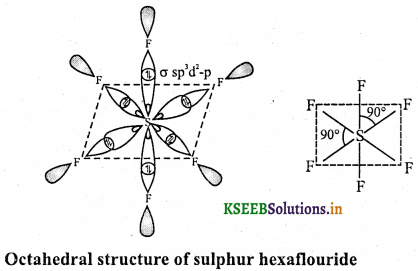Question 12.
What is the type of hybridization of carbon atoms marked with star?
(a) CH2 = CH – COOH
(b) CH3 – CH2OH
(c) CH3 – C≡CH
(a) sp2
(b) sp3
(c) sp

Molecular Orbital Theory

Question 1.
Give the salient features of molecular orbital theory (MOT).

• When two atomic orbitals having nearly same energy and proper symmetry are approaching each other, molecular orbitals are formed by the linear combination of atomic orbitals (LCAO).
• The electrons in a molecule are present in the various molecular orbitals as the electrons of atoms are present in the various atomic orbitals.
• The number of molecular orbitals formed is equal to the number of atomic orbitals undergoing combination.
• During LCAO, two types of molecular orbitals are formed,
• Bonding molecular orbitals (BMO), and
• Anti-bonding molecular orbitals (ABMO).
• Bonding molecular orbitals have less energy, more stable and favour bond formation, whereas antibonding molecular orbitals have more energy, less stable and do not favour bond formation.
• Molecular orbitals are polycentric, i.e., molecular orbitals do not associate with a particular atom but belong to the nuclei of all the atoms constituting the molecule.
• The shapes of molecular orbitals depend upon the shapes of combining atomic orbitals.
• Electrons are added to the MOs (bonding and antibonding) using the same rules that are used for adding
electrons to atomic orbitals:
• The aufbau principle, lowest energy MOs fill first.
• The Pauli exclusion principle, a maximum of two electrons per orbitals and these must be of opposite spin.
• Hund’s rule, when there are equal energy or “degenerate” orbitals, these get filled with one electron at a time before pairing begins.Question 2.
Explain the formation of molecular orbitals by linear combination of atomic orbitals (LCAO).
Linear combinations of atomic orbitals (LCAO) can be used to estimate the molecular orbitals that are formed upon bonding between the molecule’s constituent atoms. Similar to an atomic orbital, a Schrodinger equation, which describes the behaviour of an electron, can be constructed for a molecular orbital as well. Linear combinations of atomic orbitals, or the sum and difference of the atomic wave functions, provide approximate solutions to the molecular Schrodinger equations. For simple diatomic molecules, the obtained wave functions are represented mathematically by the equations
B.M.O. = σ = ΨA + ΨB
A . B . M . O = σ* = ΨA – ΨBwhere σ and σ* are the molecular orbital wavefunctions for the bonding and antibonding molecular orbitals, respectively, and ΨA and ΨB are the atomic wavefunctions for the atoms ‘A’ and ‘B’, respectively.

Thus, the molecular orbital σ formed by the addition of atomic orbitals is called the bonding molecular orbital while the molecular orbital σ* formed by the subtraction of atomic orbital is called antibonding molecular orbital.

Qualitatively, the formation of molecular orbitals can be understood in terms of the constructive or destructive interference of the electron waves of the combining atoms.

During the formation of bonding molecular orbital, the two electron waves of the bonding atoms reinforce each other due to constructive interference. As a result, the electron density in a bonding molecular orbital is located between the nuclei of the bonded atoms because of which the repulsion between the nuclei is very less. Electrons placed in a bonding molecular orbital tend to hold the nuclei together and stabilise a molecule. Therefore, a bonding molecular orbital always possesses lower energy .than either of the atomic orbitals that have combined to form it.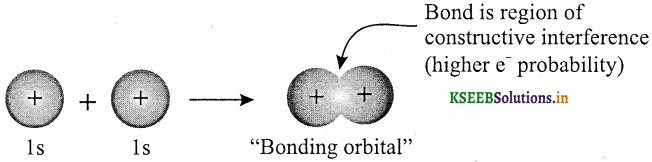During the formation of antibonding molecular orbital, the electron waves cancel each other due to destructive interference. Therefore, most of the electron density is located away from the space between the nuclei. Infact, there is a nodal plane (on which the electron density is zero) between the nuclei and hence the repulsion between the nuclei is high. The electrons placed in the antibonding molecular orbital destabilise the molecule. This is because the mutual repulsion of the electrons in this orbital is more than the attraction between the electrons and the nuclei, which causes a net increase in energy.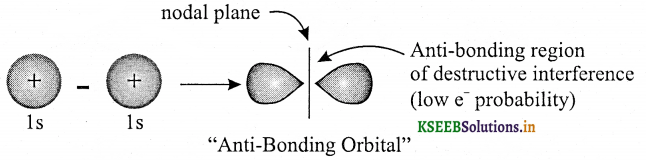Question 3.
What are the conditions for the combination of atomic orbitals.
1. The combining atomic orbitals must have same or nearly same energy. This means that Is orbital can combine with another Is orbital but not with 2s orbital. This is because Is orbital has less energy than 2s
orbital.

2. The combining atomic orbitals must have the same symmetry about the molecular axis. This means that 2px orbital of one atom can combine with 2px orbital of another atom but 2px cannot combine with 2py or 2pz because of their different symmetries.

3. The combining atomic orbitals must overlap to the maximum extent.
Note: By convention z-axis is taken as the molecular axis.

Question 69.
How many types of bonding and antibonding molecular orbitals are formed in diatomic molecules.
Two types – (i) σ and π bonding molecular orbital
(ii) σ* and π* antibonding molecular orbitals.

Question 4.
Molecular orbitals obtained from 2px and 2py orbitals are not symmetrical around the bond axis. Why?
Because of the presence of positive lobes above and below the molecular plane.
Note: π bonding MO has larger electron density above and below the inter-nuclear axis.
π* antibonding MO has a node between the nuclei.

Question 71.
Draw the shapes of molecular orbitals.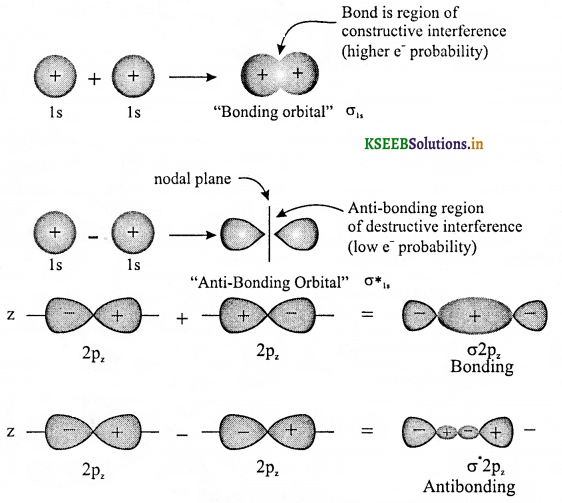Question 5.
Write the increasing order of energies of various molecular orbitals for homogeneous diatomic molecules (O2, F2) with atomic number greater than 7.
σ1s < σ*1s < σ2s < σ*2s < σ2pz < (π2px = π2py) < (π*2px = π*2py) < σ*2pz
(This order is applicable if atomic number is greater than 7, example, O2, F2)

Question 73.
Write the increasing order of energies of various molecular orbitals for homogeneous diatomic molecules (Li2  Be2, B2, C2, N2) with atomic number 7 and less than seven.
σls < σ * 1s < σ2s < σ * 2s < (π2px = π2py)< σ2pz < (π* 2px = π * 2py) < σ * 2pz
(This order is applicable if atomic number is 7 or less than 7, example, C2, N2, B2 Li2)

Question 6.
Define bond order. Give the relation between bond order, bond length, bond strength and stability.
Bond order indicates the number of covalent bonds between the two atoms. Bond order is calculated as half of the difference between the number of electrons in the bonding molecular orbitals (Nb) and the number of electrons in the antibonding molecular orbitals (Na).
Bond order = $$\frac { 1 }{ 2 }$$[Nb – Na ]
As the bond order increases, bond strength increases, bond length decreases and stability increases.

Bonding in Some Homonuclear Diatomic Molecules

Question 1.
Explain bonding in H2 molecule based on MOT.
Formation of hydrogen molecule2. Electronic configuration of H2 molecule = (σ1s)2 (σ * 1s)0
3. Molecular energy level diagram is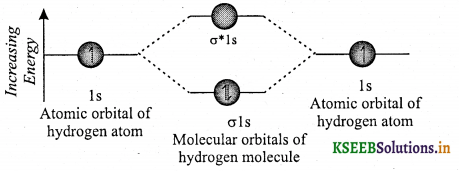Bond order $$\frac { 1 }{ 2 }$$[Nb – Na ] = $$\frac { 1 }{ 2 }$$[2 – 0] = 1
That is, the two hydrogen atoms are linked by a single covalent bond [H – H]. The bond dissociation energy of hydrogen molecule is found to be 438 kJ mol-1 and the bond length is 74 pm. H2 molecule is diamagnetic.

Question 2.
Explain non existence of helium molecule(He2) based on MOT or Helium is monoatomic. Why?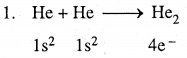2. The electronic configuration of helium molecule is He2 : (σ1s)2 (σ* 1s)2
3. Molecular orbital energy level diagram of He2 molecule is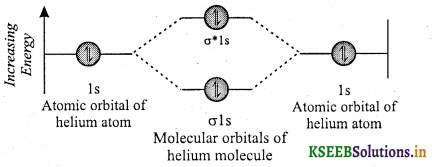Bond order = $$\frac { 1 }{ 2 }$$[Nb – Na ] = $$\frac { 1 }{ 2 }$$[2 – 2] = 0
Since bond order is zero, no bond exists between the two helium atoms. Hence helium molecule does not exist or Helium is monoatomic.Question 3.
Give the electronic configuration, bond order and magnetic property of carbon molecule based on MOT. OR Explain the formation of C2 molecule based on MOT.2. Electronic configuration of C2 molecule is
(σ1s)2s)2(σ2s)2*2s)2 (π2p2x = 2p2y) or KK(σ2S)22s)2(π2p2x = 2p2y)

3. Bond order = $$\frac { 1 }{ 2 }$$[Nb – Na ]
= $$\frac { 1 }{ 2 }$$[8 – 4] = 2
That is, the two carbon atoms are linked by two bonds [C = C]. The double bond in C2 molecule consists of both pi bonds because of the presence of four electrons in two pi molecular orbitals. Magnetic property: Since there are no unpaired electrons, carbon molecule is diamagnetic. The diamagnetic nature of C2 molecules have been detected in vapour phase.

Question 4.
Explain the formation of Li2 molecule based on MOT.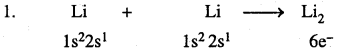2. The electronic configuration of lithium molecule σ1s2 σ*1s2 σ2s2 σ*2s°
3. Bond order = $$\frac { 1 }{ 2 }$$[Nb – Na ]
= $$\frac { 1 }{ 2 }$$[4 – 2] = 2
4. Two lithium atoms are linked by one covalent bond. (Li – Li)
5. Magnetic property: Since there are no unpaired electrons, lithium molecule is diamagnetic.

Question 5.
Explain formation of oxygen molecule (O2) based on MOT.2. Electronic configuration of 02 molecule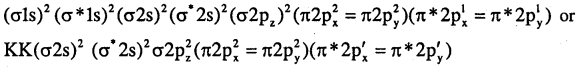3. Bond order = $$\frac { 1 }{ 2 }$$[Nb – Na ]
= $$\frac { 1 }{ 2 }$$[10 – 6] = 2
That is, two oxygen atoms are linked by two covalent bonds [O = O]
Magnetic property – oxygen molecules is paramagnetic due to the presence of 2 unpaired electrons in antibonding molecular orbitals π*2px, and π*2py

Hydrogen Bonding

Question 1.
Write a note on hydrogen bonding.
A bond formed between hydrogen atom and more electronegative atoms like F, O, N (only) is called hydrogen bond. Hydrogen bond is represented by dotted line. The length of the H-bond is more than the normal covalent bond. H-bond is weaker than normal covalent bond.
Example : Hydrogen bonding in waterHydrogen bonding in hydrogen fluoride:
——–Hδ+ _____ Fδ- ————- Hδ+ _______ Fδ- ——— Hδ+ ______ Fδ- ————-Question 2.
Explain cause for hydrogen bonding.
Answer: The hydrogen is attached directly to one of the most electronegative elements (X), causing the hydrogen to acquire a significant amount of positive charge (δ+), while ‘X’ attains a fractional negative charge (δ). This results in the formation of a polar molecule having electrostatic force of attraction, which causes hydrogen bond to form. It can be represented as:
——— Hδ+ -Xδ- ———- Hδ+ – Xδ- ——— Hδ+ -Xδ-
The magnitude of H-bonding depends on the physical state of the compound. It is maximum in the solid state and minimum in the gaseous state. Thus, the hydrogen bonds have strong influence on the structure and properties of the compounds.

Question 3.
Explain types of hydrogen bonding with examples.